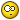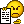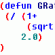Need help with a Lisp Routine

Recommended PostsI was wondering what do i need to add or modify the attach file to produce a result like this " 1@length" right now it just produces "length". I'm a novice at lisp routines but i'm learning, i can change a few things to make it do what i need it to do but this ones has gotten me stumped. Any help would be greatly appreciated.Thanks,

Brian

;Extrusion Length
(defun c:EXTL  (/ cEnt tStr tBox tHgt tWid gr sPt cPt lAng bPt tPt pt1 pt2 pt3 pt4)
(if (and (setq cEnt (car (entsel "\nSelect Object: ")))
(member (cdr (assoc 0 (entget cEnt)))
'("LWPOLYLINE" "POLYLINE" "LINE")))
(progn
(setq tStr (rtos (- (vla-get-length
(vlax-ename->vla-object cEnt)) 4.))
tBox (textbox (list (cons 1 tStr) (cons 40 (getvar "TEXTSIZE"))))
twid (- (caadr tBox) (caar tBox)))
(princ "\nPosition Text...")
(while (eq 5 (car (setq gr (grread t 5 0))))
(redraw)
(if (listp (setq sPt (cadr gr)))
(progn
(setq cPt  (vlax-curve-getClosestPointto cEnt sPt)
lAng (angle cPt sPt)
bpt  (polar cPt lAng (/ (getvar "TEXTSIZE") 2.))
tpt  (polar bpt lAng tHgt)
mPt  (polar bPt lAng (/ tHgt 2.))
pt1  (polar bpt (+ lAng (/ pi 2.)) (/ tWid 2.))
pt2  (polar bPt (- lAng (/ pi 2.)) (/ tWid 2.))
pt3  (polar tpt (+ lAng (/ pi 2.)) (/ tWid 2.))
pt4  (polar tPt (- lAng (/ pi 2.)) (/ tWid 2.)))
(grvecs (list -3 pt1 pt2 pt3 pt4 pt1 pt3 pt2 pt4)))))
(if (eq 3 (car gr))
(progn
(setq lAng (- lAng (/ pi 2.)))
(cond ((and (> lAng (/ pi 2)) (<= lAng pi))
(setq lAng (- lAng pi)))
((and (> lAng pi) (<= lAng (/ (* 3 pi) 2)))
(setq lAng (+ lAng pi))))
(Make_Text mPt tStr lAng))))
(princ "\n<!> Incorrect Selection <!>"))
(redraw)
(princ))
(defun Make_Text  (pt val rot)
(entmake
(list
(cons 0 "TEXT")
(cons 8 (getvar "CLAYER"))
(cons 62 1)
(cons 10 pt)
(cons 40 (getvar "TEXTSIZE"))
(cons 1 val)
(cons 50 rot)
(cons 7 (getvar "TEXTSTYLE"))
(cons 71 0)
(cons 72 1)
(cons 73 2)
(cons 11 pt))))

Edited by BrianTFC

Share on other sitesPlease read the CODE POSTING GUIDELINES and edit your post.Share on other sitesDo you want just to add the "1@" prefix in front of the length? For this case, please modify the code like below.

(setq tStr [color=blue](strcat "1@" [/color](rtos (- (vla-get-length
(vlax-ename->vla-object cEnt)) 4.0))[color=blue])[/color]
tBox (textbox (list (cons 1 tStr) (cons 40 (getvar "TEXTSIZE"))))
twid (- (caadr tBox) (caar tBox)))

Other, I think you need to provide more details.

Regards,

Mircea

Join the conversation

You can post now and register later. If you have an account, sign in now to post with your account.
Note: Your post will require moderator approval before it will be visible.Reply to this topic...

×   Pasted as rich text.   Paste as plain text instead

Only 75 emoji are allowed.